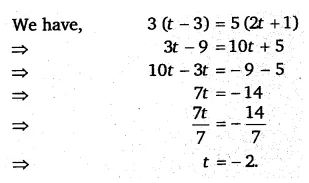# Simplify and solve the following linear equations: 3(t – 3) = 5(2t + 1)

Simplify and solve the following linear equations:
3(t – 3) = 5(2t + 1)